Ex.3.7 (Optional)

Chapter 3 Class 10 Pair of Linear Equations in Two Variables
Serial order wise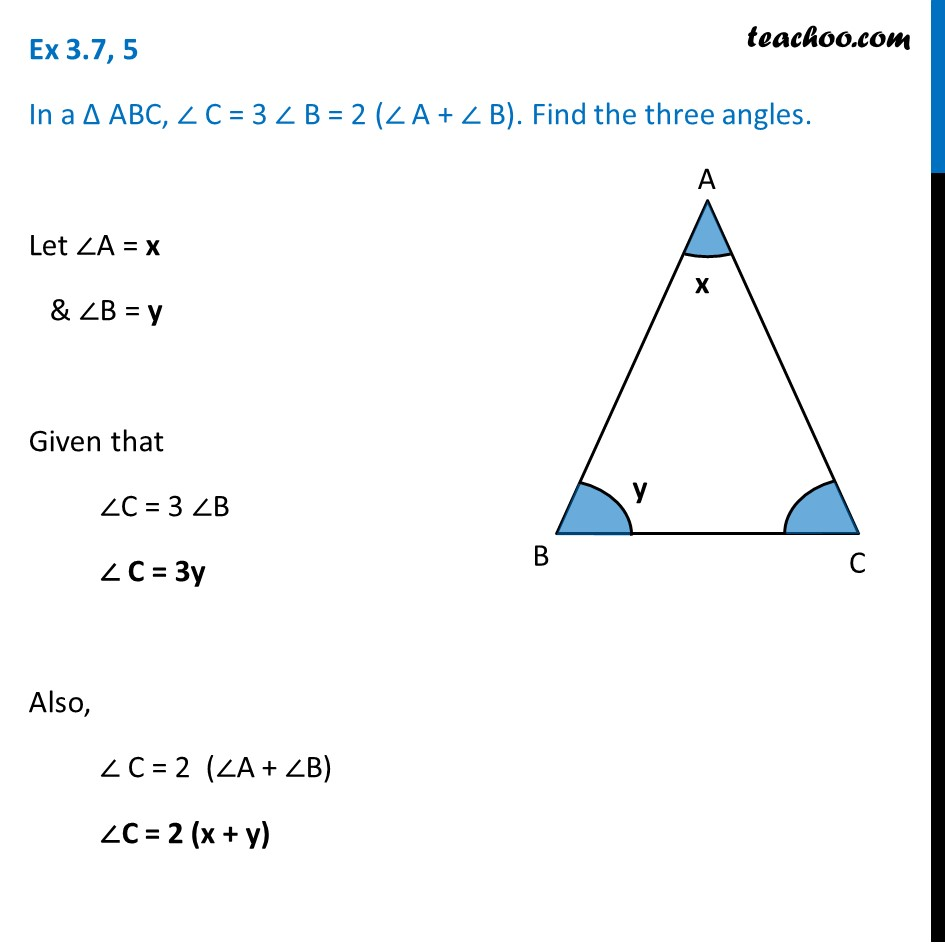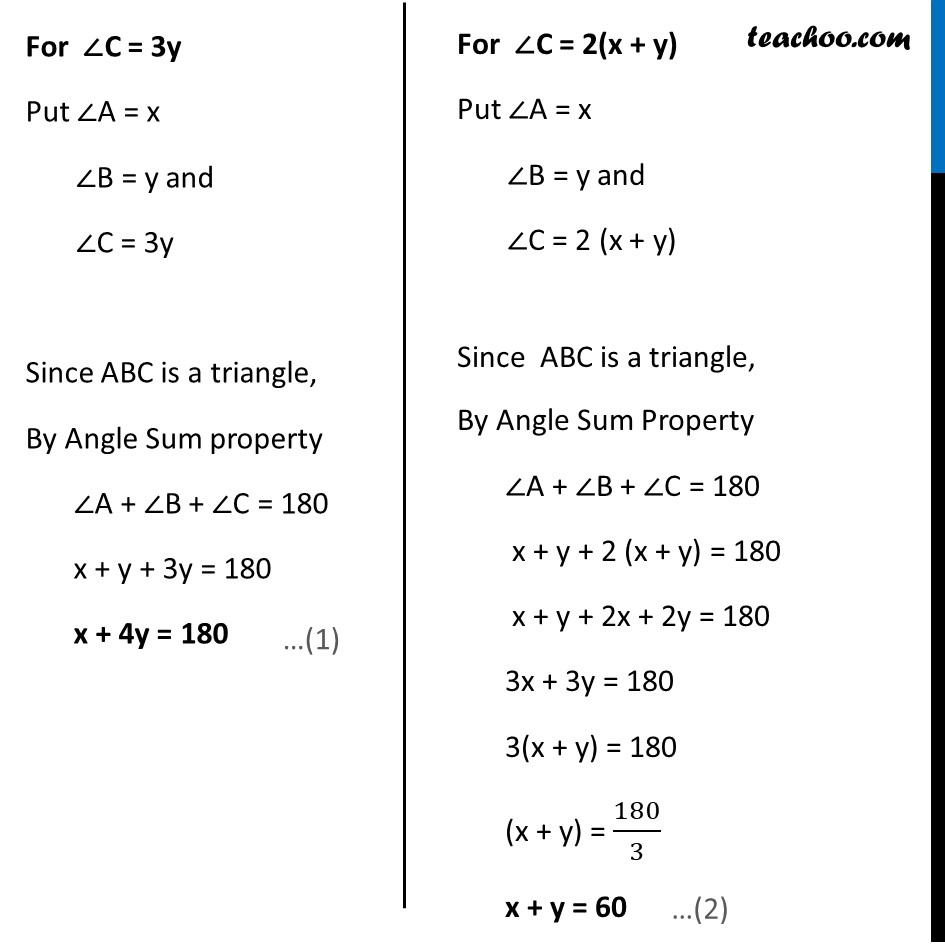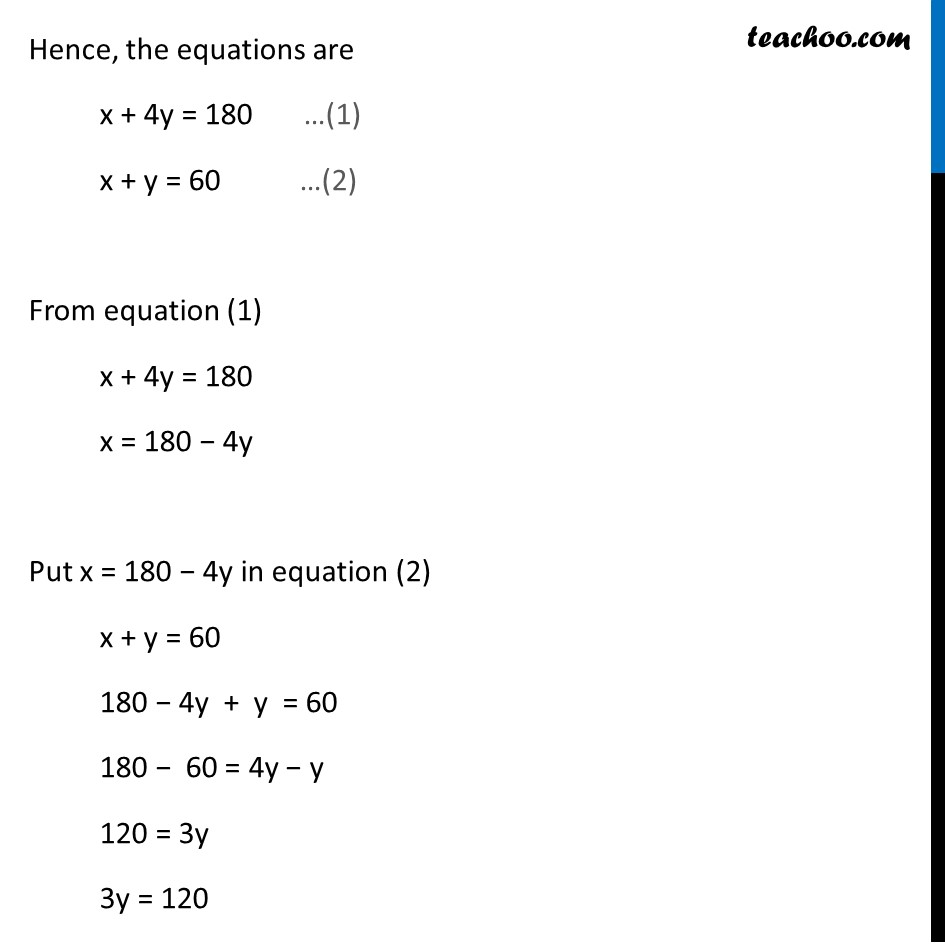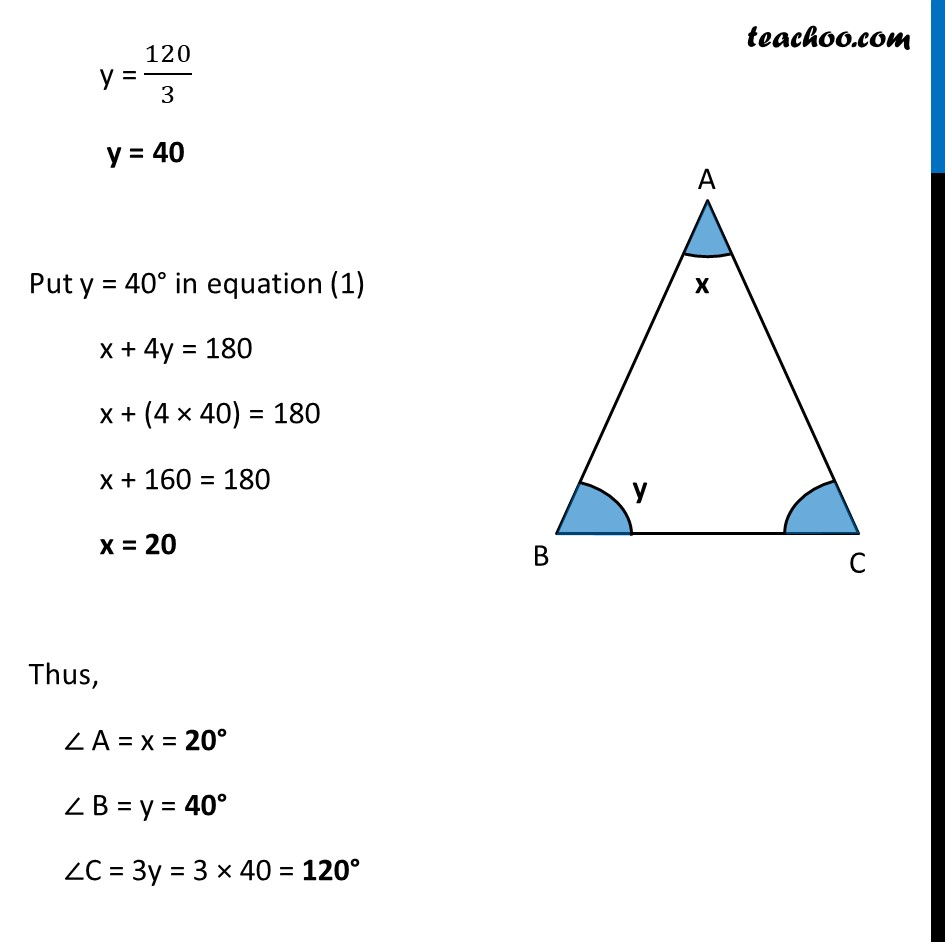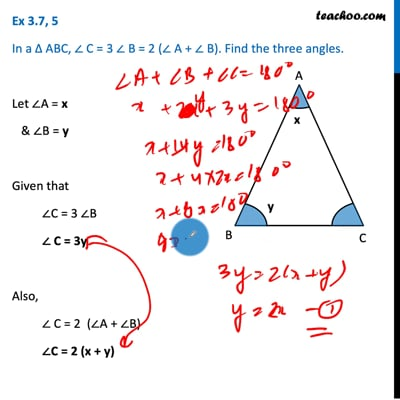This video is only available for Teachoo black users

Maths Crash Course - Live lectures + all videos + Real time Doubt solving!

### Transcript

Ex 3.7, 5 In a Δ ABC, ∠ C = 3 ∠ B = 2 (∠ A + ∠ B). Find the three angles. Let ∠A = x & ∠B = y Given that ∠C = 3 ∠B ∠ C = 3y Also, ∠ C = 2 (∠A + ∠B) ∠C = 2 (x + y) For ∠C = 3y Put ∠A = x ∠B = y and ∠C = 3y Since ABC is a triangle, By Angle Sum property ∠A + ∠B + ∠C = 180 x + y + 3y = 180 x + 4y = 180 For ∠C = 2(x + y) Put ∠A = x ∠B = y and ∠C = 2 (x + y) Since ABC is a triangle, By Angle Sum Property ∠A + ∠B + ∠C = 180 x + y + 2 (x + y) = 180 x + y + 2x + 2y = 180 3x + 3y = 180 3(x + y) = 180 (x + y) = 180/3 x + y = 60 Hence, the equations are x + 4y = 180 …(1) x + y = 60 …(2) From equation (1) x + 4y = 180 x = 180 − 4y Put x = 180 − 4y in equation (2) x + y = 60 180 − 4y + y = 60 180 − 60 = 4y − y 120 = 3y 3y = 120 y = 120/3 y = 40 Put y = 40° in equation (1) x + 4y = 180 x + (4 × 40) = 180 x + 160 = 180 x = 20 Thus, ∠ A = x = 20° ∠ B = y = 40° ∠C = 3y = 3 × 40 = 120°